# in measure the internal product attribute we use the flowgraph measurement (structure) the purpose of drawing the flowgr...

in measure the internal product attribute we use the flowgraph measurement (structure)

the purpose of drawing the flowgraph is to identify the structure of the algorithm

i need a justification of this statement (identify the structure of the algorithm)

A flowchart is the graphical or pictorial representation of an algorithm with the help of different symbols, shapes and arrows in order to demonstrate a process or a program. With algorithms, we can easily understand a program. The main purpose of a flowchart is to analyze different processes. Several standard graphics are applied in a flowchart:

There are some basic shapes and boxes included in flowcharts that are used in the structure of explaining steps of algorithms. Knowing how to use them while drawing flowcharts is crucial. Here are some rules that should be known:

1. All boxes of flowcharts are connected with arrows to show the logical connection between them,
2. Flowcharts will flow from top to bottom,
3. All flowcharts start with a Start Box and end with a Terminal Box,

Example: Calculate the Interest of a Bank Deposit

Algorithm:

• Step 4: Calculate the interest with formula "Interest=Amount*Years*Rate/100
• Step 5: Print interest,

Flowchart: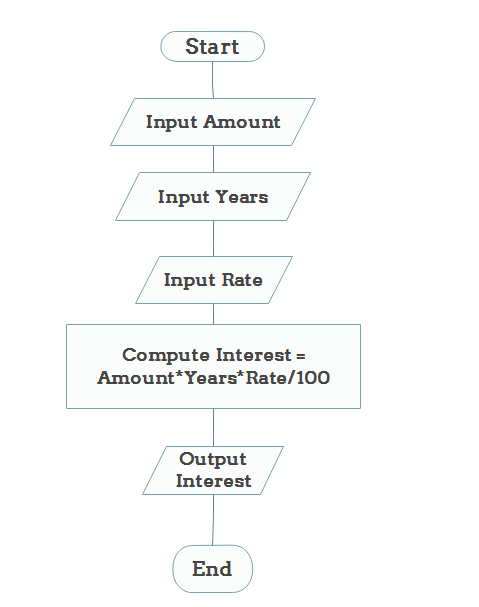​​​​​​​

##### Add Answer of: in measure the internal product attribute we use the flowgraph measurement (structure) the purpose of drawing the flowgr...
Similar Homework Help Questions
• ### 3. Suppose we measure a quantity y as a function of a parameter z. The measurement errors ony are...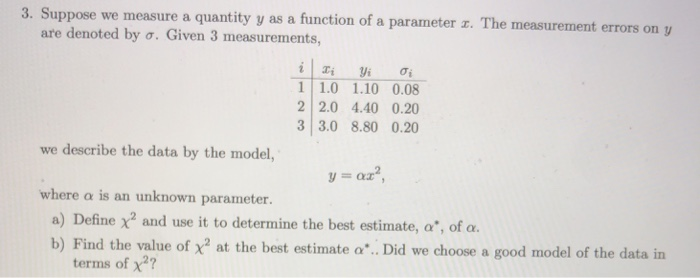3. Suppose we measure a quantity y as a function of a parameter z. The measurement errors ony are denoted by ơ. Given 3 measurements. 11.0 1.10 0.08 2 2.0 4.40 0.20 3 3.0 8.80 0.20 we describe the data by the model, where α is an unknown parameter. a) Define χ2 and use it to determine the best estimate, a", of a. b) Find the value of x2 at the best estimate a. Did we choose a good model...

• ### Use your knowledge of reaction mechanisms to predict the major product by drawing the intermeiate (or...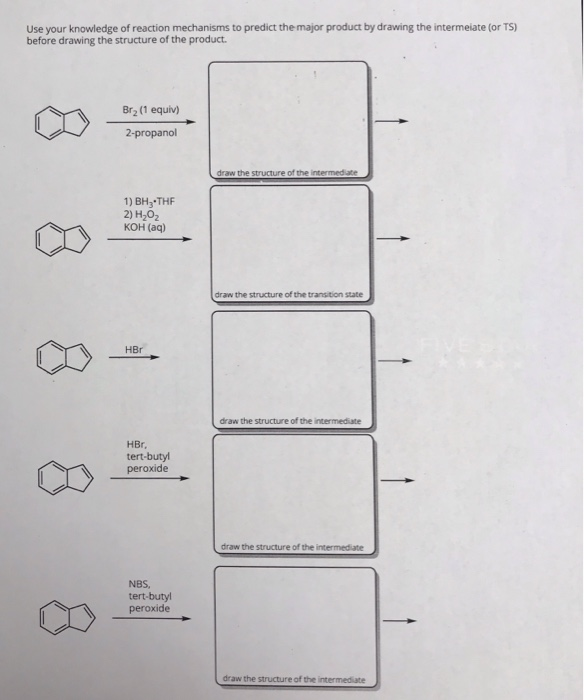Use your knowledge of reaction mechanisms to predict the major product by drawing the intermeiate (or TS) before drawing the structure of the product. 1 8 Br (1 equiv) 2-propanol draw the structure of the intermediate 1) BH3-THE 2) H202 KOH (aq) 8 draw the structure of the transition state HR 8 draw the structure of the intermediate HBr, tert-butyl peroxide 8 draw the structure of the intermediate NBS, tert-butyl peroxide 8 draw the structure of the intermediate

• ### which of the following units of metric measurement is best to use to measure the length of a bike path

which of the following units of metric measurement is best to use to measure the length of a bike path?meter,kiloliter,milligram, or kilometer i think its either meter or kilometer

• ### Click the "draw structure" button to launch the drawing utility. Draw the product of the reaction...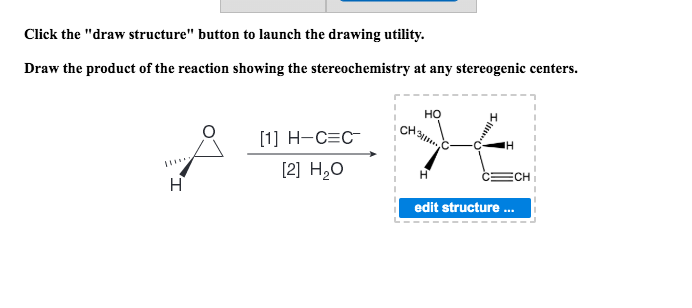Click the "draw structure" button to launch the drawing utility. Draw the product of the reaction showing the stereochemistry at any stereogenic centers. I HOH CH ....  H-C=C  H2O CECH edit structure ...

• ### Draw the structure of the product of this reaction. Use the wedge and dash tools to...Draw the structure of the product of this reaction. Use the wedge and dash tools to indicate stereochemistry of substituents on ring. Do not draw hydrogen atoms. If no reaction occurs, signify this by drawing ethane in the sketch pad.

• ### D. We will use pinv function to find a linear regression model to map the measurement data to tru...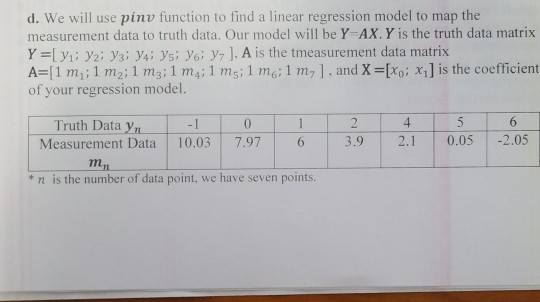d. We will use pinv function to find a linear regression model to map the measurement data to truth data. Our model will be Y-AX. Y is the truth data matrix Y [ yı; y2i yai ya; ysi yg: y7 ]. A is the tmeasurement data matrix A=[ 1 mh; 1 m2. 1 mai 1 m4; 1 ms: 1 mt 6:1 m7 I . and X-[Xo: x1] is the coefficient of your regression model. Truth Data yn -1 0 Measurement...

• ### i updated the picture Click the "draw structure" button to launch the drawing utility Draw the...i updated the picture Click the "draw structure" button to launch the drawing utility Draw the product(s) of the reaction. (Draw the polymer showing 2 repeating units and use the letter "R" on both ends to represent the remainder of polymer chain.) "OH

• ### Draw the structure of the product of this reaction. Use the wedge and dash tools to indicate stereochemistry of substit...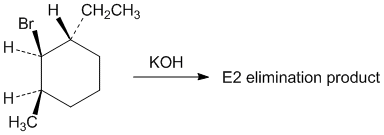Draw the structure of the product of this reaction. Use the wedge and dash tools to indicate stereochemistry of substituents on ring. Do not draw hydrogen atoms. If no reaction occurs, signify this by drawing ethane in the sketch pad.

• ### If I is the current in the external resistor R and r is the internal resistance...

If I is the current in the external resistor R and r is the internal resistance of the cell, the equation for the whole circuit is:             E = I(R + r)          …….(i)             For the same current in the external resistor R, the terminal voltage is given by:             V = IR                  …….(ii) Use equations (i) and (ii) to solve for r by eliminating I and write this equation the form: y = mx + b             Identify...

• ### Calorimetry is the measurement of heat transfer. Devices that measure the amount of heat released or...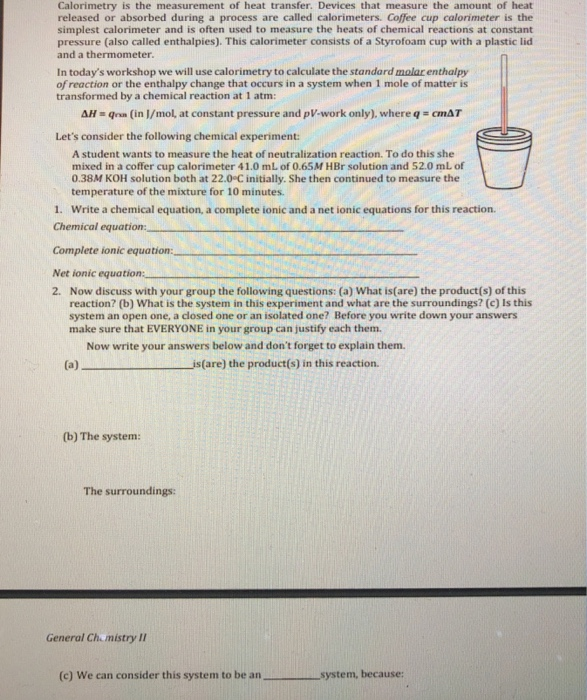Calorimetry is the measurement of heat transfer. Devices that measure the amount of heat released or absorbed during a process are called calorimeters. Coffee cup calorimeter is the simplest calorimeter and is often used to measure the heats of chemical reactions at constant pressure (also called enthalpies). This calorimeter consists of a Styrofoam cup with a plastic lid and a thermometer In today's workshop we will use calorimetry to calculate the standard molar enthalpy of reaction or the enthalpy change...

Need Online Homework Help?